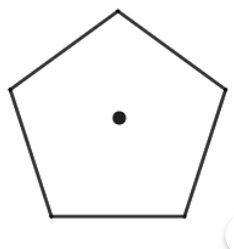What is the order of the rotational symmetry of the given figure?

# What is the order of the rotational symmetry of the given figure?1. A
4
2. B
3
3. C
8
4. D
5

Fill Out the Form for Expert Academic Guidance!l

+91

Live ClassesBooksTest SeriesSelf Learning

Verify OTP Code (required)

### Solution:

Concept- Apply the fact that an n-sided regular polygon's rotational symmetry order is always equal to n.
The figure is given asLet us just examine rotational symmetry in order to explain this question.
When an object is rotated (turned) a certain number of degrees about its center, it is said to have rotational symmetry.
The number of positions an object appears the same in a 360o rotation is the order of symmetry.
For the given figures, there are 5 rotational symmetry orders.
Hence, the correct answer is option (4).

## Related content

 Area of Square Area of Isosceles Triangle Pythagoras Theorem Triangle Formula Perimeter of Triangle Formula Area Formulae Volume of Cone Formula Matrices and Determinants_mathematics Critical Points Solved Examples Type of relations_mathematics+91

Live ClassesBooksTest SeriesSelf Learning

Verify OTP Code (required)